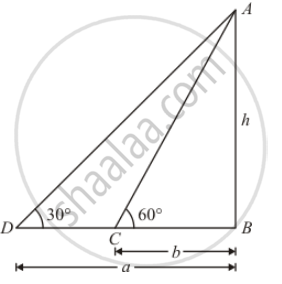Advertisement Remove all ads

# If the Angles of Elevation of a Tower from Two Points Distant a and B (A>B) from Its Foot and in the Same Straight Line from It Are 30° and 60°, Then the Height of the Tower is - Mathematics

MCQ

If the angles of elevation of a tower from two points distant a and b (a>b) from its foot and in the same straight line from it are 30° and 60°, then the height  of the tower is

#### Options

• $\sqrt{a + b}$

• $\sqrt{ab}$

• $\sqrt{a - b}$

• $\sqrt{\frac{a}{b}}$

Advertisement Remove all ads

#### Solution

Let h be the height of tower ABGiven that: angle of elevation are∠C=60° and∠D=60° .∠D=30°

Distance BC=b  andBD=a

Here, we have to find the height of tower.

So we use trigonometric ratios.

In a triangle ABC,

⇒ tan C=( AB)/(BC)

⇒ tan 60°=(AB)/(BC)

⇒ tan 60°= h/b

Again in a triangle ABD

⇒ tan D=(AB)/(BD)

⇒ tan 30°=h/a

Again in a triangle ABD,

⇒ tan D=(AB)/(BD)

⇒ tan 30°=h/a

⇒ tan (90°-60°)=h/a

⇒ cot 60°=h/a

⇒ 1/tan 60°=h/a

⇒ b/h=h/a              "put tan "60°=h/b

⇒ h^2=ab

⇒h=ab

h=sqrt(ab)

Is there an error in this question or solution?
Advertisement Remove all ads

#### APPEARS IN

RD Sharma Class 10 Maths
Chapter 12 Trigonometry
Q 4 | Page 41
Advertisement Remove all ads

#### Video TutorialsVIEW ALL 

Advertisement Remove all ads
Share
Notifications

View all notifications

Forgot password?
Course